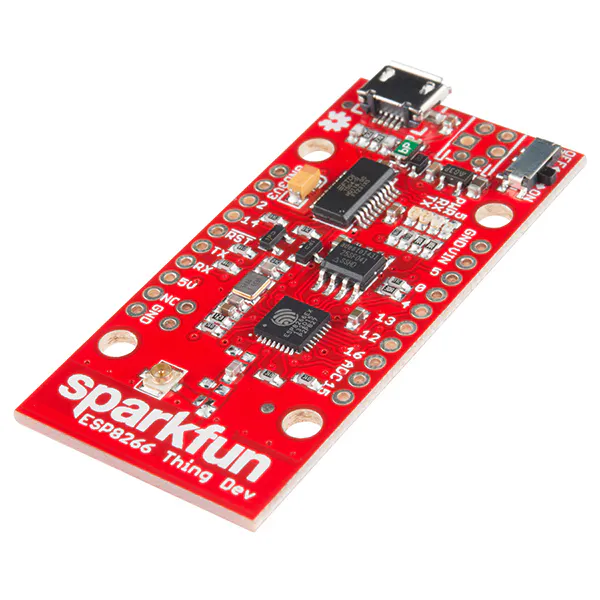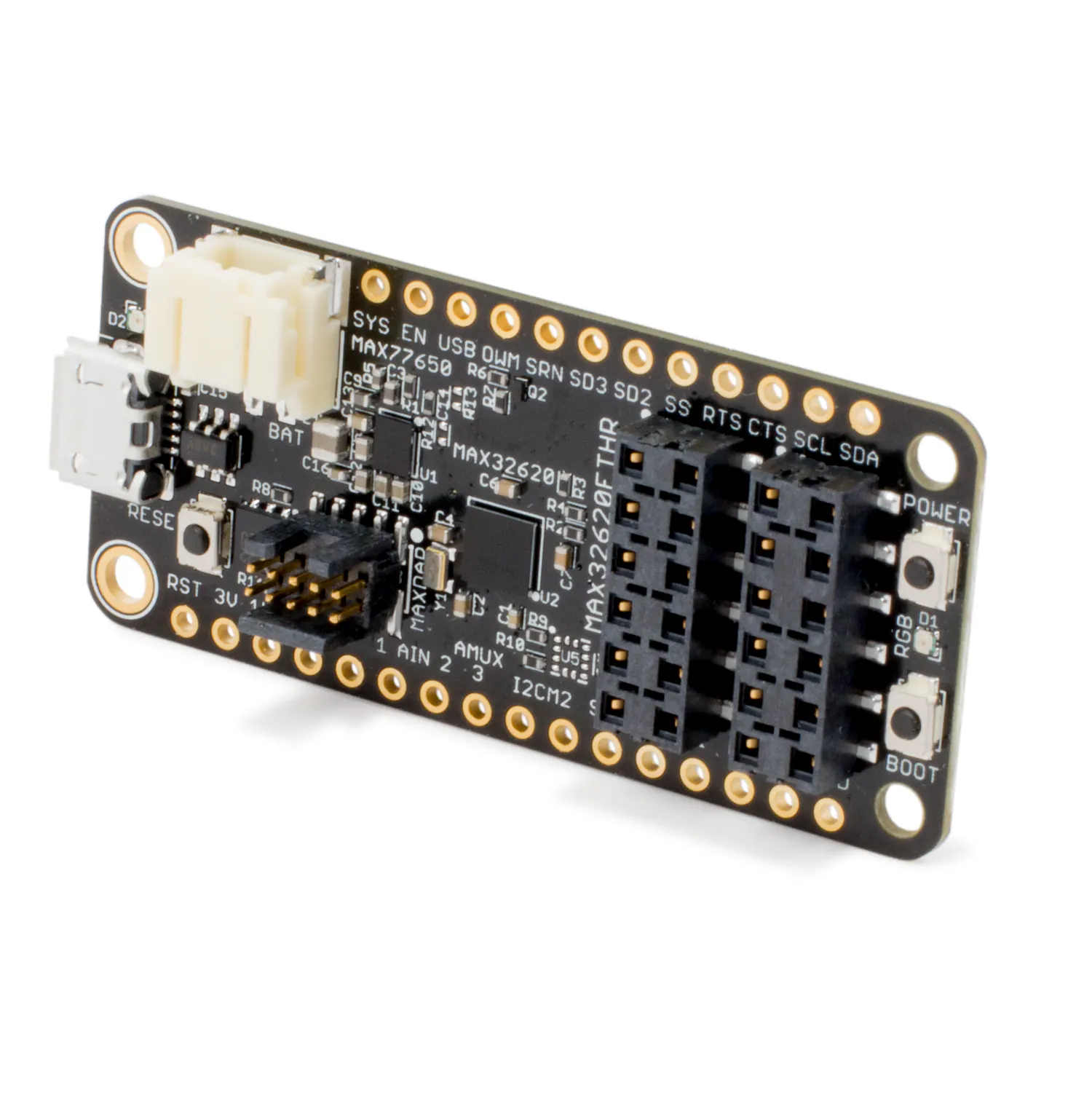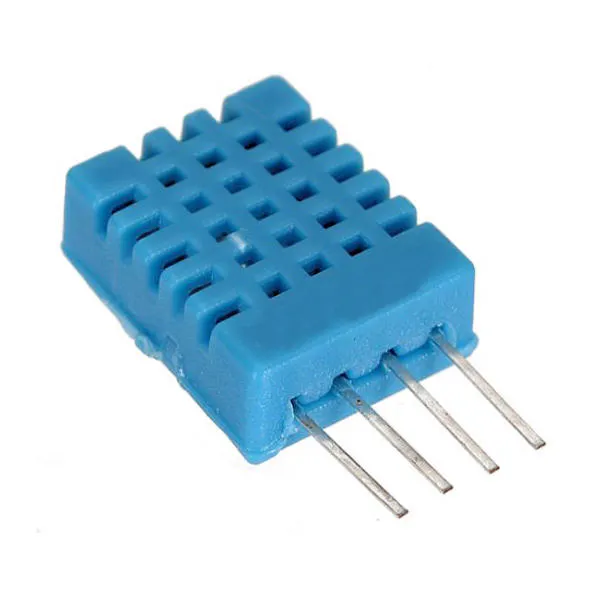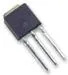# MAX32620FTHR IoT Aquaponics Systems

Smart system that'll help you eat fresh vegetables and fish with low water consumption and zero fertilizers, even if you don't have garden.

## Things used in this project

### Hardware componentsSparkFun ESP8266 Thing - Dev Board
×1Maxim Integrated MAX32620FTHR
×1DHT11 Temperature & Humidity Sensor (4 pins)
×1Ultrasonic Sensor - HC-SR04 (Generic)
×1Power MOSFET N-Channel
×3
 Temperature probe (Generic)
×1
 Relay Module (Generic)
×1LED (generic)
×1
 5M SMD 3528 300 Waterproof LED RGB Strip Flexible Light 24 key IR remote + Power Adapter DC12V
×1
 DC motor (generic)
×1

### Software apps and online servicesUnityArduino IDE

### Hand tools and fabrication machines

 screw
 wood saw

## Code

### sensors (water temp , Ldr , Soil Moisture)

Arduino
```// the setup routine runs once when you press reset:
void setup() {
// initialize serial communication at 9600 bits per second:
Serial.begin(9600);
}

// the loop routine runs over and over again forever:
void loop() {
//soil moisture 800 out of water , 300 inside water
// read the input on analog pin 0:
// map it to the range of the analog out:
int  outputValue = 150;
// change the analog out value:
analogWrite(47, outputValue);

// print out the value you read:

Serial.print("soil moisture : ");
Serial.print(sensorValue1);
Serial.print(",");
// print out the value you read:
Serial.print("temprature : ");
Serial.print(sensorValue2);
Serial.print(",");

// print the results to the Serial Monitor:
Serial.print("light sensor = ");
Serial.print(sensorValue);
Serial.print("\t input voltage = ");
Serial.println(outputValue);

delay(5000);
}
```

### test sending and receiving sparkfun

Arduino
```#include <ESP8266WiFi.h>
#include <FirebaseArduino.h>

// Set these to run example
#define FIREBASE_HOST "FIREBASE DATABASE LINK " // Formated like that without http or / (aquaponicsystem-9e0ec.firebaseio.com")
#define FIREBASE_AUTH "FIREBASE DATABASE AUTH "
#define WIFI_SSID "WIFI NAME"

xPin, sendTxPin);

String actuator = "";
void setup() {
// Begin the Serial at 9600 Baud
Serial.begin(9600);
// mySerial.begin(9600);
//  mySerial2.begin(9600);
// connect to wifi.
// Serial.print("connecting");
while (WiFi.status() != WL_CONNECTED) {
//   Serial.print(".");
delay(500);
}
Firebase.begin(FIREBASE_HOST, FIREBASE_AUTH);

}
void loop() {
String data = "";
//-------- get sensor reeading from maxfthr board ---------//
if (Serial.available()) {
data = String(Serial.readStringUntil('\n'));             // sends ascii code
delay (1000);
char *str;
char sz ;
data.toCharArray(sz, 50);
char *p = sz;
String words ;
int i = 0 ;
while (str = strtok (p, ","))
{
words[i] = str;
//Serial.println(str);
p = NULL;
i = i + 1 ;
}

delay(1000);
if (words != 0 && words != 0 && words != 0 && words != 0 && words != 0 && words != 0 )
{

Firebase.setString("airhumidity", words);
Firebase.setString("airtemp", words);
Firebase.setString("light", words);
Firebase.setString("watertemp", words);
Firebase.setString("soilmoisture", words);
Firebase.setString("waterlevel", words);
delay(5000); //Wait for the serial port.
}
}
else
{
Serial.print("serial1 not available ");
//   Firebase.setString("recieveddata", "error");
delay(1500); //Wait for the serial
}
Firebase.setString("recieveddata", data);

//------ get actuators state from firebase-------//
String red = "" , green = "" , blue = "" ;
float feeder = 0.00 , heater = 0.00 , pump = 0.00 ;
red = Firebase.getString("red");
delay(100);
green = Firebase.getString("green");
delay(100);
blue = Firebase.getString("blue");
delay(100);
feeder = Firebase.getFloat("feederstate");
delay(100);
heater = Firebase.getFloat("heaterstate");
delay(1000);
pump = Firebase.getFloat("pumpstate");
delay(100);

//------------- send values to maxfthr board -------------//
actuator = "" ;
actuator.concat(red);
actuator.concat(",");
actuator.concat(green);
actuator.concat(",");
actuator.concat(blue);
actuator.concat(",");
actuator.concat(feeder);
actuator.concat(",");
actuator.concat(heater);
actuator.concat(",");
actuator.concat(pump);
actuator.concat(",");
Firebase.setString("actuator", actuator);

if (feeder == 1.00 || heater == 1.00 || pump == 1.00)
{
//if state of any of heater , feeder , pump is on set it's value as 1 and       send this value to max fthr
//and wait 1 minuite or any time u want and then turn it off again by setting   value to zero
Serial.println(actuator);
delay (5000);
Firebase.setFloat("feederstate", 0.00);
Firebase.setFloat("heaterstate",  0.00);
Firebase.setFloat("pumpstate", 0.00);
}
Serial.println(actuator);
actuator = "";

delay (5000);
}
```

### test send and receive max

Arduino
```void setup() {
//==================================//
//    Serial Communication          //
//==================================//
Serial.begin (9600);
Serial2.begin(9600);
}
void loop() {
//         Print all                //
Serial2.println("218,72,132,0.00,0.00,0.00,20,25,2"); //Write the serial data
delay (1000);

if (Serial2.available()) {
String data = String(Serial2.readStringUntil('\n'));             // sends ascii code
Serial.print("READING DATA FROM SERIAL 2 :  ");
Serial.println(data); //Print data on Serial Monitor
char *str;
char sz ;
data.toCharArray(sz, 50);
char *p = sz;
String words = "";
int i = 0 ;
while (str = strtok (p, ","))
{
words[i] = str;
//Serial.println(str);
p = NULL;
i = i + 1 ;
}

Serial.print("Red : ");
Serial.print(words);
Serial.print("Green : ");
Serial.print(words);
Serial.print("Blue : ");
Serial.print(words);
Serial.print("Heater : ");
Serial.print(words);
Serial.print("Pump : ");
Serial.print(words);
Serial.print("Feeder : ");
Serial.print(words);
Serial.println("");
delay(1500);
} else
{
Serial.print("serial 2 not available ");
delay(5000); //Wait for the serial
}
}
```

### MaxFthr Full Code

Arduino
```/*
Smart Aquaponic Project

*/

int DHpin = 28;
byte dat ;
byte data;
for (int i = 0; i < 8; i ++) {
if (digitalRead (DHpin) == LOW) {
while (digitalRead (DHpin) == LOW); // wait for 50us
delayMicroseconds (30); // determine the duration of the high level to determine the data is '0 'or '1'
data |= (1 << (7-i)); // high front and low in the post
while (digitalRead (DHpin) == HIGH); // data '1 ', wait for the next one receiver
}
}
return data;
}

void start_test () {
digitalWrite (DHpin, LOW); // bus down, send start signal
delay (30); // delay greater than 18ms, so DHT11 start signal can be detected

digitalWrite (DHpin, HIGH);
delayMicroseconds (40); // Wait for DHT11 response

pinMode (DHpin, INPUT);
delayMicroseconds (80); // DHT11 response, pulled the bus 80us
delayMicroseconds (80); // DHT11 80us after the bus pulled to start sending data

for (int i = 0; i < 4; i ++) // receive temperature and humidity data, the parity bit is not considered

pinMode (DHpin, OUTPUT);
digitalWrite (DHpin, HIGH); // send data once after releasing the bus, wait for the host to open the next Start signal
}

// defines pins numbers
const int trigPin = 26;
const int echoPin = 29;
// defines variables
long duration;
int distance;

int Red = 40;
int Green = 41;
int Blue = 42;// the PWM pin the LED is attached to

void setup () {
Serial.begin (9600);
Serial2.begin(9600);
pinMode (DHpin, OUTPUT);

pinMode(trigPin, OUTPUT); // Sets the trigPin as an Output
pinMode(echoPin, INPUT); // Sets the echoPin as an Input

pinMode(Red, OUTPUT);
pinMode(Green, OUTPUT);
pinMode(Blue, OUTPUT);

pinMode(27, OUTPUT);
pinMode(43, OUTPUT);
pinMode(44, OUTPUT);
}

void loop() {
// Clears the trigPin
digitalWrite(trigPin, LOW);
delayMicroseconds(2);
// Sets the trigPin on HIGH state for 10 micro seconds
digitalWrite(trigPin, HIGH);
delayMicroseconds(10);
digitalWrite(trigPin, LOW);
// Reads the echoPin, returns the sound wave travel time in microseconds
duration = pulseIn(echoPin, HIGH);
// Calculating the distance
distance= duration*0.034/2;
// Prints the distance on the Serial Monitor
Serial.print("Distance: ");
Serial.println(distance);

//soil moisture 800 out of water , 300 inside water
// read the input on analog pin 0:
// map it to the range of the analog out:
int  outputValue = 150;
// change the analog out value:
analogWrite(47, outputValue);

// print out the value you read:

Serial.print("soil moisture : ");
Serial.print(sensorValue1);
Serial.print(",");
// print out the value you read:
Serial.print("temprature : ");
Serial.print(sensorValue2);
Serial.print(",");

// print the results to the Serial Monitor:
Serial.print("light sensor = ");
Serial.print(sensorValue);
Serial.print("\t input voltage = ");
Serial.println(outputValue);

delay(5000);

//         Print all                //

delay (1000);

if (Serial2.available()) {
String data = String(Serial2.readStringUntil('\n'));             // sends ascii code
Serial.print("READING DATA FROM SERIAL 2 :  ");
Serial.println(data); //Print data on Serial Monitor
char *str;
char sz ;
data.toCharArray(sz, 50);
char *p = sz;
String words = "";
int i = 0 ;
while (str = strtok (p, ","))
{
words[i] = str;
//Serial.println(str);
p = NULL;
i = i + 1 ;
}

analogWrite(Red, words.toInt());
analogWrite(Green, words.toInt());
analogWrite(Blue, words.toInt());
analogWrite(20, words.toInt());
delay(5000);

digitalWrite(27, words.toInt());   // turn the LED on (HIGH is the voltage level)
delay(1000);                       // wait for a second
digitalWrite(43, words.toInt());   // turn the LED on (HIGH is the voltage level)
delay(1000);                       // wait for a second
digitalWrite(44, words.toInt());   // turn the LED on (HIGH is the voltage level)
delay(1000);                       // wait for a second

Serial.print("Red : ");
Serial.print(words);
Serial.print("Green : ");
Serial.print(words);
Serial.print("Blue : ");
Serial.print(words);
Serial.print("Heater : ");
Serial.print(words);
Serial.print("Pump : ");
Serial.print(words);
Serial.print("Feeder : ");
Serial.print(words);
Serial.println("");
delay(1500);
} else
{
Serial.print("serial 2 not available ");
delay(5000); //Wait for the serial
}

}
```

### thing dev full code

Arduino
```#include <ESP8266WiFi.h>
#include <FirebaseArduino.h>

// Set these to run example
#define FIREBASE_HOST "FIREBASE DATABASE LINK " // Formated like that without http or / (aquaponicsystem-9e0ec.firebaseio.com")
#define FIREBASE_AUTH "FIREBASE DATABASE AUTH "
#define WIFI_SSID "WIFI NAME"

String actuator = "";
void setup() {
// Begin the Serial at 9600 Baud
Serial.begin(9600);
while (WiFi.status() != WL_CONNECTED) {
delay(500);
}
Firebase.begin(FIREBASE_HOST, FIREBASE_AUTH);
}
void loop() {
String data = "";
//-------- get sensor reeading from maxfthr board ---------//
if (Serial.available()) {
data = String(Serial.readStringUntil('\n'));             // sends ascii code
delay (1000);
char *str;
char sz ;
//   String testData = "18,72,132,0.00,218,72,132,0.00,0.00,0.00";
data.toCharArray(sz, 50);
char *p = sz;
String words ;
int i = 0 ;
while (str = strtok (p, ","))
{
words[i] = str;
p = NULL;
i = i + 1 ;
}
// set value
delay(1000);
if (words != 0 && words != 0 && words != 0 && words != 0 && words != 0 && words != 0 )
{
Firebase.setString("airhumidity", words);
Firebase.setString("airtemp", words);
Firebase.setString("light", words);
Firebase.setString("watertemp", words);
Firebase.setString("soilmoisture", words);
Firebase.setString("waterlevel", words);
delay(5000); //Wait for the serial port.
}
}
else
{
Serial.print("serial1 not available ");
//   Firebase.setString("recieveddata", "error");
delay(1500); //Wait for the serial
}
Firebase.setString("recieveddata", data);
//------ get actuators state from firebase-------//
String red = "" , green = "" , blue = "" ;
float feeder = 0.00 , heater = 0.00 , pump = 0.00 ;
red = Firebase.getString("red");
delay(100);
green = Firebase.getString("green");
delay(100);
blue = Firebase.getString("blue");
delay(100);
feeder = Firebase.getFloat("feederstate");
delay(100);
heater = Firebase.getFloat("heaterstate");
delay(1000);
pump = Firebase.getFloat("pumpstate");
delay(100);
//------------- send values to maxfthr board -------------//
actuator = "" ;
actuator.concat(red);
actuator.concat(",");
actuator.concat(green);
actuator.concat(",");
actuator.concat(blue);
actuator.concat(",");
actuator.concat(feeder);
actuator.concat(",");
actuator.concat(heater);
actuator.concat(",");
actuator.concat(pump);
actuator.concat(",");
actuator.concat(0);
actuator.concat(",");
Firebase.setString("actuator", actuator);
if (feeder == 1.00 || heater == 1.00 || pump == 1.00)
{
//if state of any of heater , feeder , pump is on set it's value as 1 and send this value to max fthr
//and wait 1 minuite or any time u want and then turn it off again by setting value to zero
Serial.println(actuator);
delay (5000);
Firebase.setFloat("feederstate", 0.00);
Firebase.setFloat("heaterstate",  0.00);
Firebase.setFloat("pumpstate", 0.00);
}
Serial.println(actuator);
actuator = "";
delay (5000);
}
```

### Ultrasonic sensor

Arduino
```/*
* Ultrasonic Sensor HC-SR04 and Arduino Tutorial
*
* by Dejan Nedelkovski,
* www.HowToMechatronics.com
*
*/
// defines pins numbers
const int trigPin = 26;
const int echoPin = 29;
// defines variables
long duration;
int distance;
void setup() {
pinMode(trigPin, OUTPUT); // Sets the trigPin as an Output
pinMode(echoPin, INPUT); // Sets the echoPin as an Input
Serial.begin(9600); // Starts the serial communication
}
void loop() {
// Clears the trigPin
digitalWrite(trigPin, LOW);
delayMicroseconds(2);
// Sets the trigPin on HIGH state for 10 micro seconds
digitalWrite(trigPin, HIGH);
delayMicroseconds(10);
digitalWrite(trigPin, LOW);
// Reads the echoPin, returns the sound wave travel time in microseconds
duration = pulseIn(echoPin, HIGH);
// Calculating the distance
distance= duration*0.034/2;
// Prints the distance on the Serial Monitor
Serial.print("Distance: ");
Serial.println(distance);
}
```

### Led strip

Arduino
```int Red = 40;
int Green = 41;
int Blue = 42;// the PWM pin the LED is attached to
// the setup routine runs once when you press reset:
void setup() {
// declare pins to be an output:
pinMode(Red, OUTPUT);
pinMode(Green, OUTPUT);
pinMode(Blue, OUTPUT);
}
// the loop routine runs over and over again forever:
void loop() {
// set the brightness of pin 9:
analogWrite(Red, 0);
analogWrite(Green, 0);
analogWrite(Blue, 0);
analogWrite(20, 0);
delay(5000);
analogWrite(Red, 100);
analogWrite(Green, 100);
analogWrite(Blue, 100);
analogWrite(20, 100);
delay(5000);
analogWrite(Red, 254);
analogWrite(Green, 254);
analogWrite(Blue, 254);
analogWrite(20, 254);
delay(5000);
}
```

### Relays Test

Arduino
```void setup() {
// initialize digital pin LED_BUILTIN as an output.
pinMode(27, OUTPUT);
pinMode(43, OUTPUT);
pinMode(44, OUTPUT);
}

// the loop function runs over and over again forever
void loop() {
digitalWrite(27, HIGH);   // turn the LED on (HIGH is the voltage level)
delay(1000);                       // wait for a second
digitalWrite(43, HIGH);   // turn the LED on (HIGH is the voltage level)
delay(1000);                       // wait for a second
digitalWrite(44, HIGH);   // turn the LED on (HIGH is the voltage level)
delay(1000);                       // wait for a second
digitalWrite(27, LOW);    // turn the LED off by making the voltage LOW
delay(1000);                       // wait for a second
digitalWrite(43, LOW);    // turn the LED off by making the voltage LOW
delay(1000);                       // wait for a second
digitalWrite(44, LOW);    // turn the LED off by making the voltage LOW
delay(1000);                       // wait for a second
}
```

### DHT11 Temperature & humidity sensor

Arduino
```int DHpin = 28;
byte dat ;
byte data;
for (int i = 0; i < 8; i ++) {
if (digitalRead (DHpin) == LOW) {
while (digitalRead (DHpin) == LOW); // wait for 50us
delayMicroseconds (30); // determine the duration of the high level to determine the data is '0 'or '1'
data |= (1 << (7-i)); // high front and low in the post
while (digitalRead (DHpin) == HIGH); // data '1 ', wait for the next one receiver
}
}
return data;
}

void start_test () {
digitalWrite (DHpin, LOW); // bus down, send start signal
delay (30); // delay greater than 18ms, so DHT11 start signal can be detected

digitalWrite (DHpin, HIGH);
delayMicroseconds (40); // Wait for DHT11 response

pinMode (DHpin, INPUT);
delayMicroseconds (80); // DHT11 response, pulled the bus 80us
delayMicroseconds (80); // DHT11 80us after the bus pulled to start sending data

for (int i = 0; i < 4; i ++) // receive temperature and humidity data, the parity bit is not considered

pinMode (DHpin, OUTPUT);
digitalWrite (DHpin, HIGH); // send data once after releasing the bus, wait for the host to open the next Start signal
}

void setup () {
Serial.begin (9600);
pinMode (DHpin, OUTPUT);
}

void loop () {
start_test ();
Serial.print ("Current humdity =");
Serial.print (dat , DEC); // display the humidity-bit integer;
Serial.print ('.');
Serial.print (dat , DEC); // display the humidity decimal places;
Serial.println ('%');
Serial.print ("Current temperature =");
Serial.print (dat , DEC); // display the temperature of integer bits;
Serial.print ('.');
Serial.print (dat , DEC); // display the temperature of decimal places;
Serial.println ('C');
delay (700);
}
```